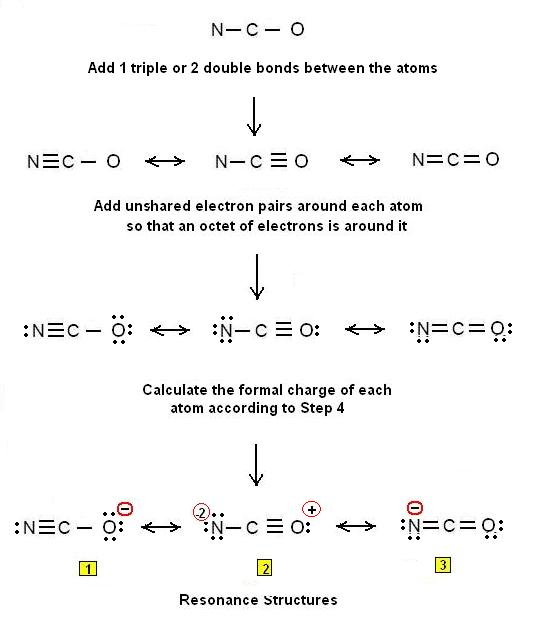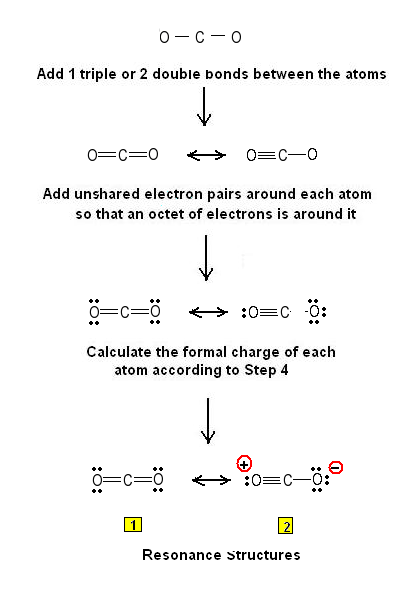Simple Procedure for writing Lewis Structures- CO2, NCO- – Examples #2 | Chemistry Net

# Simple Procedure for writing Lewis Structures- CO2, NCO- – Examples #2

Simple Method for writing Lewis Structures- CO2, NCO-

# Simple Method for writing Lewis Structures - CO2, NCO-

A simple method for writing Lewis electron dot structures is given in a previous article entitled “Lewis Structures and the Octet Rule”. Examples for writing Lewis structures following the above procedure are given bellow: Consider the case of the Lewis electron dot structures of the NCO- (cyanate ion):

Step 1: The central atom will be the C atom since it is the less electronegative.  Connect the C atom with the N and O atoms with single bonds

Step 2: Calculate the # of electrons in π bonds (pi bonds, multiple bonds) using formula (1) in the article entitled “Lewis Structures and the Octet Rule”. Where n in this case is 3 since NCO-  consists of three atoms.

Where V = (5 + 4 + 6) – (-1)  = 16   Therefore, P = 6n + 2 – V = 6 * 3 + 2 – 16 = 4  There are 4 π electrons (pi electrons) in NCO-   and therefore 2 double   bonds must be added to the structure of Step 1 or 1 triple bond.

Step 3 & 4: One double bond between C and O is added to the structure in step 1 and a second double bond between C and N. Alternatively, 1 triple bond is added either between C and O or between C and N. Unshared electron pairs are added so that there is an octet of electrons around each atom. All the equivalent resonance structures are drawn by delocalizing electron pairs. Therefore, the Lewis structures for NCO- are as follows:Figure I.1: Lewis structures for the cyanate ion NCO-. Because of the extreme charge separation in the resonance form 2, it is considered that it does not contribute much to the ground state of the molecule. Resonance forms 1 and 3 are equivalent and contribute to the ground state of the molecule – there is less charge separation in them in comparison to resonance form 2. The above Lewis structures indicate that the cyanate ion is capable of bonding at either the nitrogen or oxygen to a metal atom, as indeed is known to occur.

Let us consider the case of the Lewis electron dot structures of carbon dioxide CO2:

Step 1: The central atom will be the C atom since it is the less electronegative.  Connect the C atom with the O atoms with single bonds

Step 2: Calculate the # of electrons in π bonds (pi bond s, multiple bonds) using  formula(1): Where n in this case is 3 since CO2 consists of three atoms. Where V = (6 + 4 + 6) = 16   Therefore, P = 6n + 2 – V = 6 * 3 + 2 – 16 = 4     There are 4 π electrons inCO2 and therefore 2 double bonds must be added to the structure of Step 1 or 1 triple bond.

Step 3 & 4: Two double bonds between C and O are added to the structure in step 1. Alternatively, 1 triple bond is added between C and O. Unshared electron pairs are added so that there is an octet of electrons around each atom. All the equivalent resonance structures of CO2 are drawn by delocalizing electron pairs. Therefore, the Lewis structures for CO2 are as follows:Figure I.2: Lewis structures for the CO2 molecule. Resonance form 1 contributes significantly to the ground state of the molecule. Resonance form 2 is not significant – it is not energetically favored – because of the larger charge separation compared to 1.

Please see also the following video explanation of how to draw the Lewis dot structure of CO2:

Relevant Posts

1. G.N. Lewis, J.A.C.S, 38, 762-785, (1916)
2.  E. C. McGoran, J. Chem. Educ., 68, 19-23 (1991)
3. A.B.P. Lever, J. Chem. Educ., 49, 819-821, (1972)

Key Terms

Lewis structures of cyanate, NCO-, electron dot structures of carbon dioxide, Lewis electron dot structure of CO2